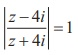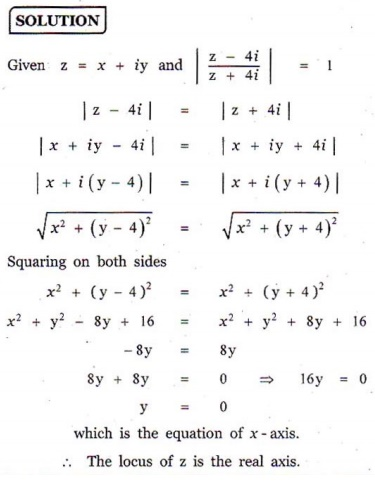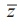Home | | Maths 12th Std | Exercise 2.6: Geometry and Locus of Complex Numbers

# Exercise 2.6: Geometry and Locus of Complex Numbers

Maths Book back answers and solution for Exercise questions - Mathematics : Complex Numbers: Geometry and Locus of Complex Numbers: Problem Questions with Answer, Solution

EXERCISE 2.6

1. If iy is a complex number such thatshow that the locus of is real axis.2. If iy is a complex number such that Im= 0 , show that the locus of is

2x2 + 2 y2 + - 2 = 0.3. Obtain the Cartesian form of the locus of iy in each of the following cases:

(i)  [Re (iz )]2 = 3

(ii) Im[(1- i)z +1] = 0

(iii) |z + i| = |z -1|

(iv)= z-1.4. Show that the following equations represent a circle, and, find its centre and radius.

(i) |z - 2 â€“ i| = 3

(ii) |2z + 2 - 4i| = 2

(iii) |3z - 6 +12i| = 85. Obtain the Cartesian equation for the locus of iy in each of the following cases:

(i)  |z â€“ 4| = 16

(ii) |z â€“ 4|2 - |z -1|2 = 16 .